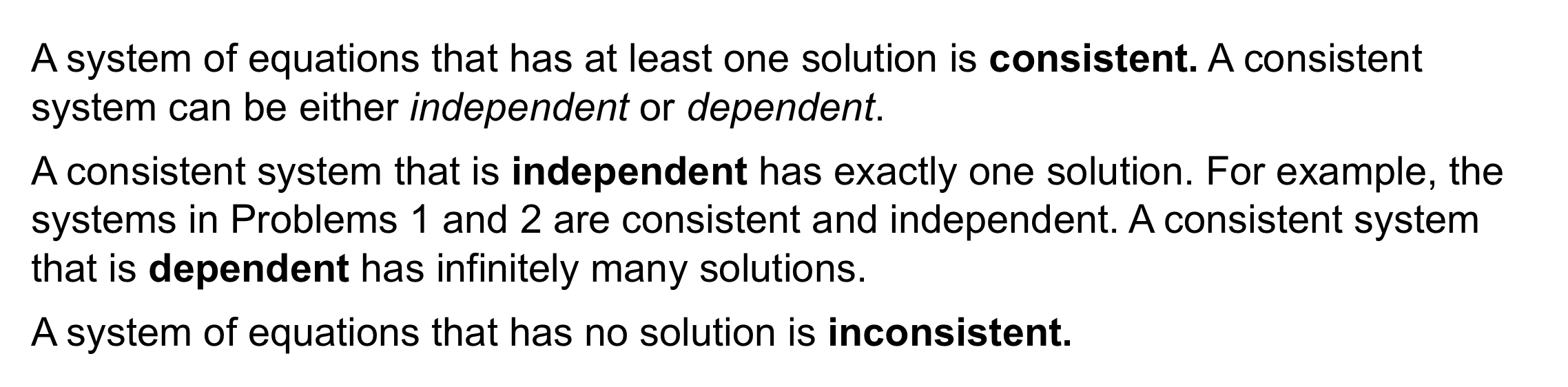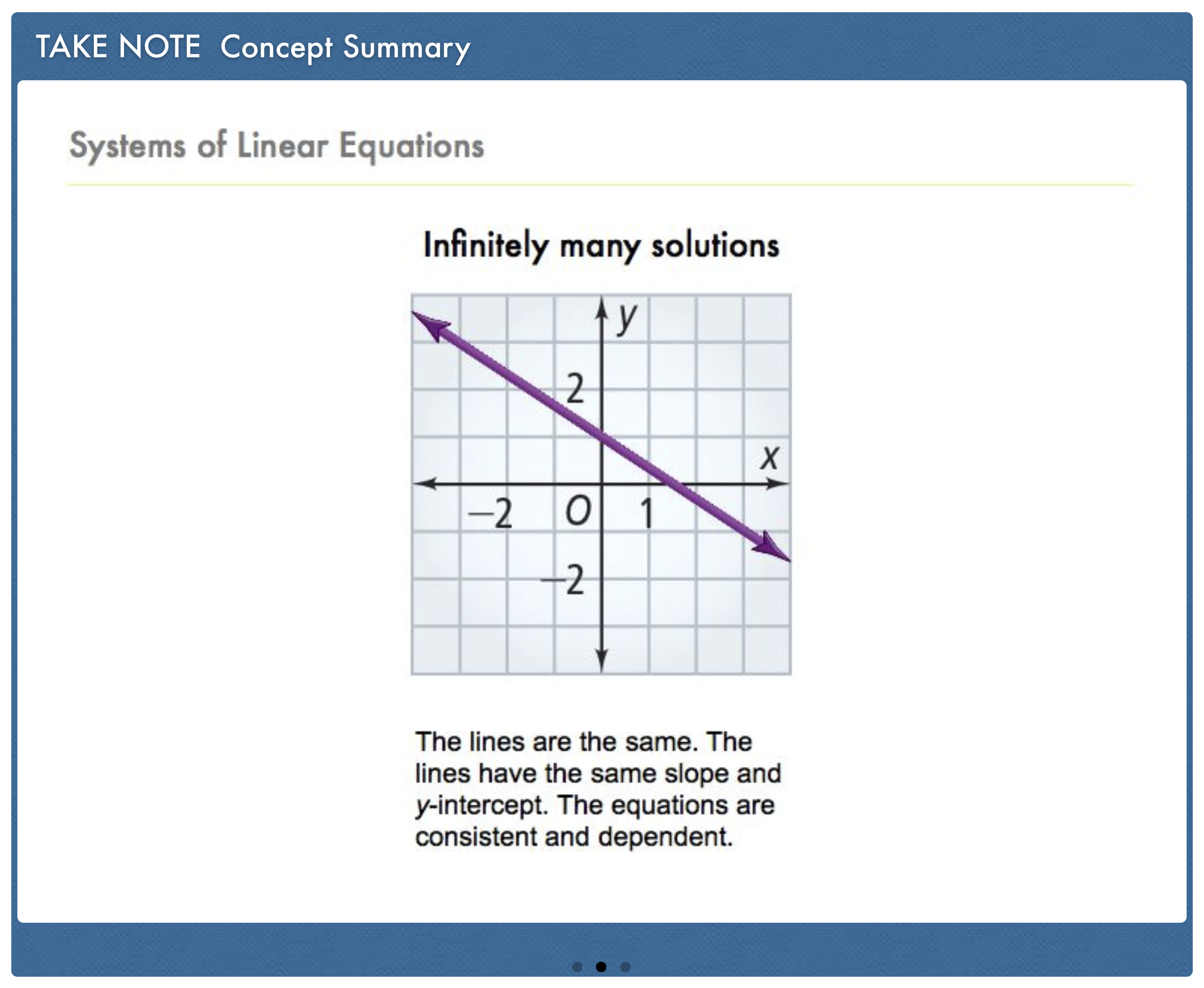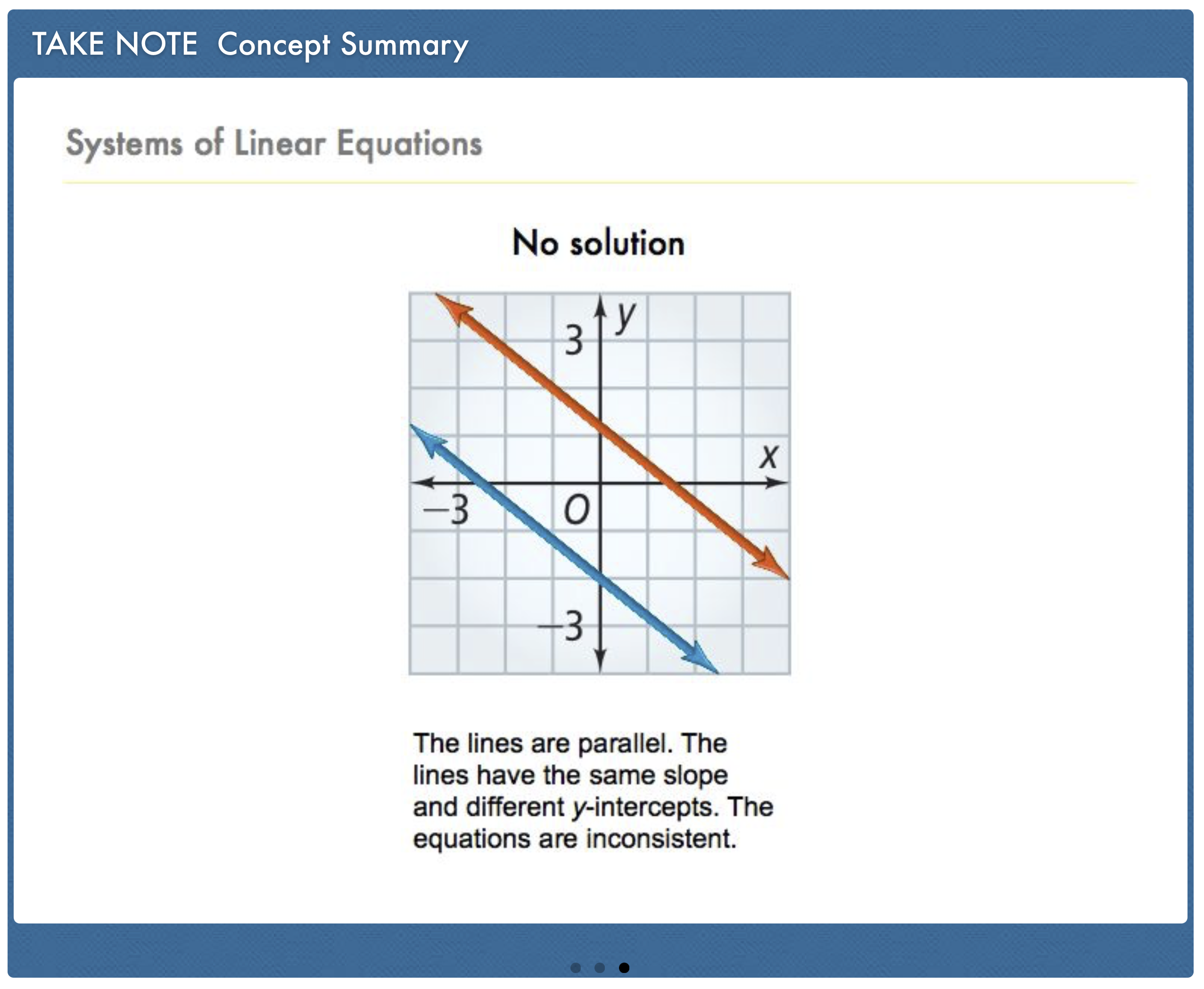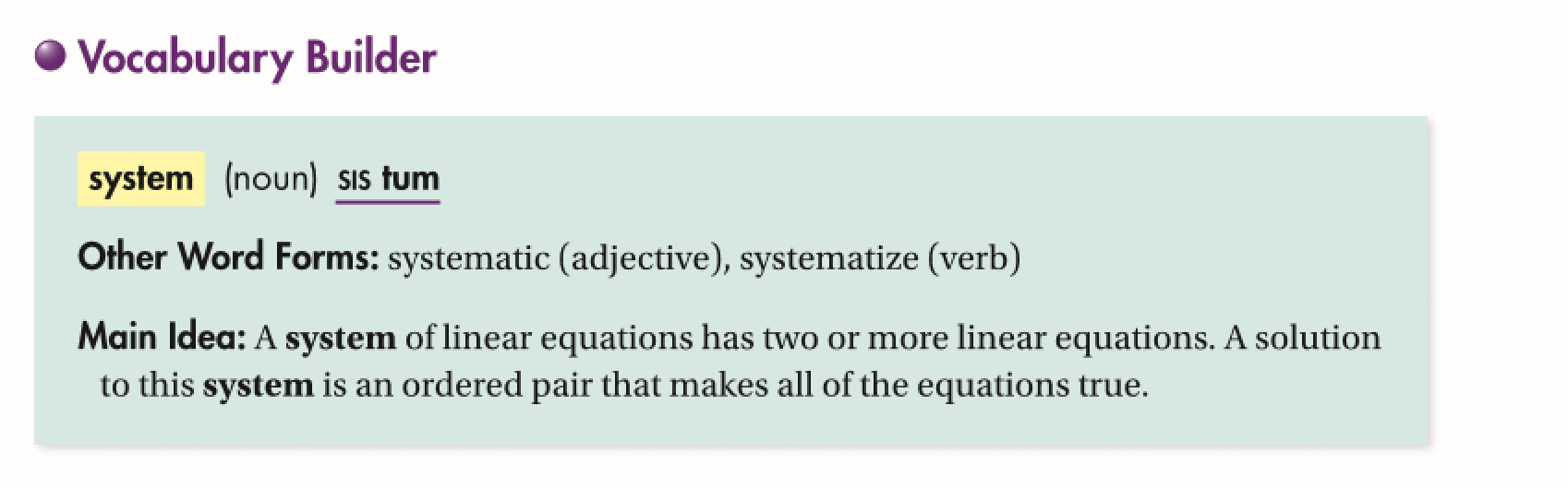Algebra 1 6-1 Complete Lesson: Solving Systems by Graphing
starstarstarstarstarstarstarstarstarstar
4.5 (1 rating)
by Matthew Richardson
| 34 Questions
Note from the author:
A complete formative lesson with embedded slideshow, mini lecture screencasts, checks for understanding, practice items, mixed review, and reflection. I create these assignments to supplement each lesson of Pearson's Common Core Edition Algebra 1, Algebra 2, and Geometry courses. See also mathquest.net and twitter.com/mathquestEDU.The outlined content above was added from outside of Formative.1
2
1
5 pts
Solve It! Two professional downhill skiers are racing at the speeds shown in the diagram. Skier 1 starts 5 s before Skier 2. The course is 5000 ft long. Will Skier 2 pass Skier 1?
Yes
No
2
10 pts
Solve It! How do you know?3
3
10 pts
Problem 1 Got It?
A
B
C
D4
4
10 pts
Problem 2 Got It?
A
B
C
D5
10 pts
Problem 3 Got It? Identify the solution(s) of the system by graphing.
Be sure to include relevant graph detail: label axes, indicate units and scale on both axes, and use arrows to represent end behavior, as appropriate.
6
10 pts
Problem 3 Got It? Identify the solution(s) of the system by graphing.
Be sure to include relevant graph detail: label axes, indicate units and scale on both axes, and use arrows to represent end behavior, as appropriate.
7
10 pts
Problem 3 Got It? Reasoning: Before graphing the equations, how can you determine whether a system of equations has exactly one solution, infinitely many solutions, or no solution?Complete all of the graphing in this assignment by hand before checking your work using the embedded Desmos graphing calculator below. After checking your work, you may edit your own graphs.
The outlined content above was added from outside of Formative.
8
20 pts
Solve the system by graphing.
Work carefully and precisely to ensure that you graphs reveal the correct solution.
As always, check your solution using substitution.

9
10 pts
Identify the solution to the system of equations you solved by graphing in the previous item.
Write your response in the following format, with a space immediately following the comma: (5, -4)
(0, 7)
(-3, 7)
(6, 13)
(2, 5)
10
20 pts
Solve the system by graphing.
Work carefully and precisely to ensure that you graphs reveal the correct solution.
As always, check your solution using substitution.

11
10 pts
Identify the solution to the system of equations you solved by graphing in the previous item.
Write your response in the following format, with a space immediately following the comma: (5, -4)
12
20 pts
Solve the system by graphing.
Work carefully and precisely to ensure that you graphs reveal the correct solution.
As always, check your solution using substitution.

13
10 pts
Identify the solution to the system of equations you solved by graphing in the previous item.
Write your response in the following format, with a space immediately following the comma: (5, -4)
14
20 pts
Solve the system by graphing. Zoom and pan your graph to establish an appropriate viewing window.

15
10 pts
Identify the solution to the system of equations you solved by graphing in the previous item.
Write your response in the following format, with a space immediately following the comma: (5, -4)
16
40 pts
Concert Tickets: Tickets for a concert cost \$10 each if you order them online, but you must pay a service charge of \$8 per order. The tickets are \$12 each if you buy them at the door on the night of the concert, with no service charge.

a. Write a system of equations to model the situation. Let c be the total cost. Let t be the number of tickets.

b. Graph the equations and find the intersection point.
17
10 pts
Analysis: What does the intersection point of the system of equations you graphed in number 9 represent?18
5 pts
Vocabulary: How many solutions does an inconsistent system have?
exactly one
no solutions
infinitely many
19
5 pts
Vocabulary: How many solutions does a consistent and dependent system have?
infinitely many
no solutions
exactly one
20
5 pts
Vocabulary: How many solutions does an consistent and independent system have?
exactly one
infinitely many
no solutions
21
10 pts
Writing: Suppose you graph a system of linear equations. If a point is on only one of the lines, is it a solution of the system? Explain.
Yes; As long as the point is on at least one line, it is a solution of the system.
No; A point must be on both lines to be a solution of the system.
22
10 pts
Reasoning: Can a system of two linear equations have exactly two solutions? Explain.
No; Two lines can not intesect in exactly two places. They must intersect in 0, 1, or infinitely-many places (aka overlap one another). This means that a system of linear equations must have 0, 1, or infinitely-many solutions.
Yes; Two lines may intersect at exactly two places. This means that a system of equations may have exactly 2 solutions.
23
10 pts
Reasoning: Suppose you find that two linear equations are true when x = -2 and y = 3. What can you conclude about the graphs of the equations? Explain.
You can conclude that the graphs of the linear equations will have at least 2 points of intersection.
You can conclude that the graphs of the linear equations will share the common point (-2, 3).
You can conclude that one of the graphs is a horizontal line and that the other is a vertical line.24
10 pts
Review Lesson 5-8: Graph the functions by translating the graph of y = |x|. Graph with colors that match the functions.
25
10 pts
Review Lesson 5-6: Find the slope of a line that is parallel to the graph of the equation.

-1
1
3
-3
26
5 pts
Review Lesson 5-6: Find the slope of a line that is parallel to the graph of the equation.

4
2
-1/4
-1/2
27
5 pts
Review Lesson 5-6: Find the slope of a line that is parallel to the graph of the equation.

-2
3/2
-2/3
2/7
28
10 pts
Review Lesson 2-5: Solve the equation for y.

y = 2x +38
y = -2x + 19
y = 2x + 19
y = -2x + 38
29
10 pts
Review Lesson 2-5: Solve the equation for y.

y = (8/15)x
y = x
y = (4/3)x
y = (6/5)x30
10 pts
Vocabulary Review: Label each amount described as infinite or finite.
• infinite
• finite
• the rational numbers greater than 6
• the number of seats in a movie theater
• the number of grams in one kilogram
• the set of odd numbers31
10 pts
Use Your Vocabulary: Complete each sentence with the appropriate word from the list.
• systematic
• system
• systematize
• The librarian planned to __?__ the donated magazines.
• The American __?__ of government is based on the Constitution.
• Sam was __?__ in his approach to studying for his final exam.
• A __?__ of linear equations might consist of two equations.
The outlined content above was added from outside of Formative.
32
10 pts
Vocabulary: Use the embedded Desmos graphing utility above to graph and take screenshots of three systems of linear equations. One must have no solutions, one must have exactly 1 solution, and one must have infinitely many solutions. Add your screenshots to the respective area of the canvas.
33
100 pts
Notes: Take a clear picture or screenshot of your Cornell notes for this lesson. Upload it to the canvas. Zoom and pan as needed.

For a refresher on the Cornell note-taking system, click here.
34
10 pts
Reflection: Math Success
Add to my formatives list

Formative uses cookies to allow us to better understand how the site is used. By continuing to use this site, you consent to the Terms of Service and Privacy Policy.